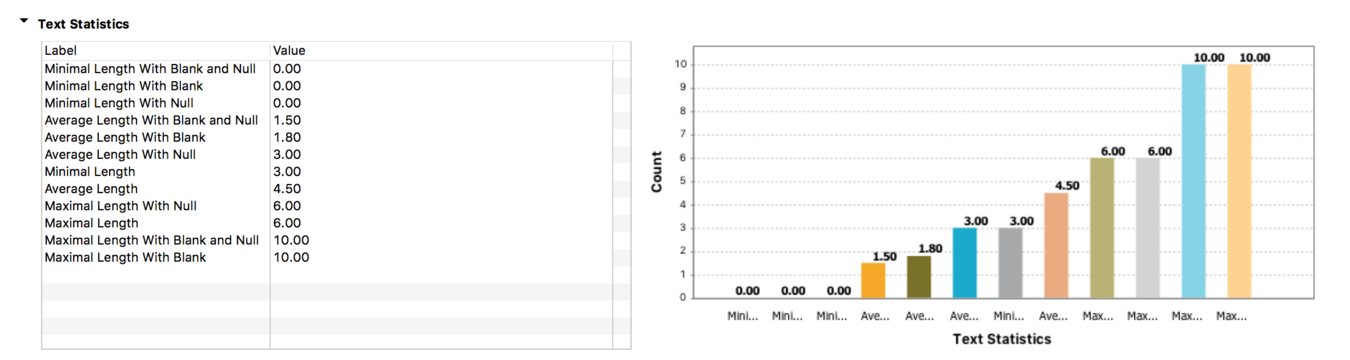Indicator types - 6.5

Talend Open Studio for MDM User Guide

EnrichVersion
6.5
EnrichProdName
Talend Open Studio for MDM
Data Governance
Data Quality and Preparation
Design and Development
EnrichPlatform
Talend Studio

Two types of indicators are listed under the Indicators folder in the DQ Repository tree view: system indicators and user-defined indicators.

User-defined indicators, as their name indicates, are indicators created by the user. You can use them through a simple drag-and-drop operation from the User Defined Indicators folder in the tree view. User-defined indicators are used only with column analyses. For more information on how to set user-defined indicators for columns, see How to set user-defined indicators from the analysis editor.

System indicators are predefined indicators grouped under different categories in the System Indicators folder in the DQ Repository tree view. Each category of the system indicators is used with a corresponding analysis type.

You can not create a system indicator or drag it directly from the DQ Repository tree view to an analysis. However, you can open and modify the parameters of a system indicator to adapt it to a specific database for example. For further information, see How to edit a system indicator.

Only system indicators you can modify are listed under the System Indicators folder in the DQ Repository tree view. However, the [Indicator Selection] dialog box lists all indicators including the system indicators you can not modify, such as Date pattern frequency.

Several management options including editing, duplicating, importing and exporting are possible for system and user-defined indicators. For more information, see Managing user-defined indicators and Managing system indicators.

The following sections to follow describe the system indicators used only on column analyses. These system indicators can range from simple or advanced statistics to text strings analysis, including summary data and statistical distributions of records.

They determine the most probable and the most frequent values and builds frequency tables. The main advanced statistics include the following values:

• Mode: computes the most probable value. For numerical data or continuous data, you can set bins in the parameters of this indicator. It is different from the "average" and the "median". It is good for addressing categorical attributes.

• Value Frequency: computes the number of most frequent values for each distinct record.

• All other Value Frequency indicators are available to aggregate date and numerical data (with respect to "date", "week", "month", "quarter", "year" and "bin").

• Value Low Frequency: computes the number of less frequent records for each distinct record.

• All other Value Low Frequency indicators are available to aggregate date and numerical data (with respect to "date", "week", "month", "quarter", "year" and "bin"), where "bin" is the aggregation of numerical data by intervals.

Fraud Detection

The Benford Law indicator (first-digit law) is based on examining the actual frequency of the digits 1 through 9 in numerical data. It is usually used as an indicator of accounting and expenses fraud in lists or tables.

Benford's law states that in lists and tables the digit 1 tends to occur as a leading digit about 30% of the time. Larger digits occur as the leading digits with lower frequency, for example the digit 2 about 17%, the digit 3 about 12% and so on. Valid, unaltered data will follow this expected frequency. A simple comparison of first-digit frequency distribution from the data you analyze with the expected distribution according to Benford's law ought to show up any anomalous results.

For example, let's assume an employee has committed fraud by creating and sending payments to a fictitious vendor. Since the amounts of these fictitious payments are made up rather than occurring naturally, the leading digit distribution of all fictitious and valid transactions (mixed together) will no longer follow Benford's law. Furthermore, assume many of these fraudulent payments have 2 as the leading digit, such as 29, 232 or 2,187. By using the Benford Law indicator to analyze such data, you should see the amounts that have the leading digit 2 occur more frequently than the usual occurrence pattern of 17%.

When using the Benford Law indicator, it is advised to:

• make sure that the numerical data you analyze do not start with 0 as Benford's law expects the leading digit to range only from 1 to 9. This can be verified by using the number > Integer values pattern on the column you analyze.

• check the order of magnitude of the data either by selecting the min and max value indicators or by using the Order of Magnitude indicator you can import from Talend Exchange. This is because Benford's law tends to be most accurate when values are distributed across multiple orders of magnitude. For further information about importing indicators from Talend Exchange, see How to import user-defined indicators from Talend Exchange.

In the result chart of the Benford Law indicator, digits 1 through 9 are represented by bars and the height of the bar is the percentage of the first-digit frequency distribution of the analyzed data. The dots represent the expected first-digit frequency distribution according to Benford's law.

Below is an example of the results of an analysis after using the Benford Law indicator and the Order of Magnitude user-defined indicator on a total_sales column.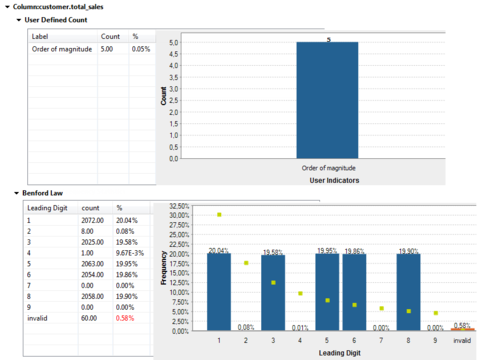The first chart shows that the analyzed data varies over 5 orders of magnitude, that is there are 5 digits between the minimal value and maximal value of the numerical column.

The second chart shows that the actual distribution of the data (height of bars) does not follow the Benford's law (dot values). The differences are very big between the frequency distribution of the sales figures and the expected distribution according to Benford's law. For example, the usual occurrence pattern for sales figures that start with 1 is 30% and those figures in the analyzed data represent only 20%. Some fraud could be suspected here, sales figures may have been modified by someone or some data may be missing.

Below is another example of the result chart of a column analysis after using the Benford Law indicator.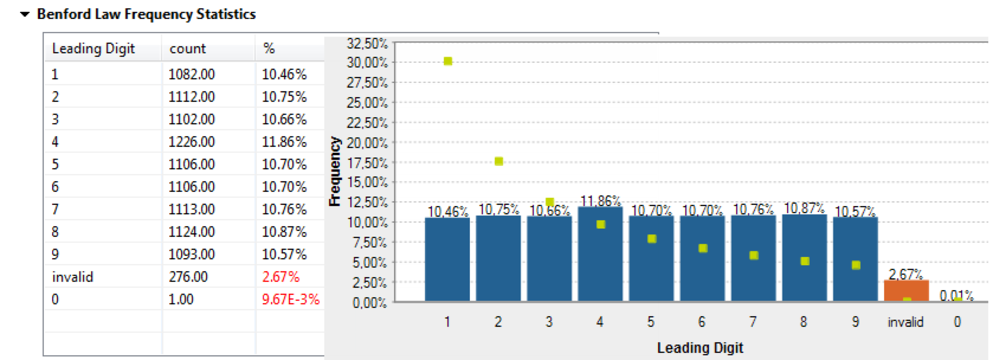The red bar labeled as invalid means that this percentage of the analyzed data does not start with a digit. And the 0 bar represents the percentage of data that starts with 0. Both cases are not expected when analyzing columns using the Benford Law indicator and this is why they are represented in red.

For further information about analyzing columns, see Creating a basic analysis on a database column.

Pattern frequency statistics

Indicators in this group determine the most and less frequent patterns.

• Pattern Frequency: computes the number of most frequent records for each distinct pattern.

• Pattern Low Frequency: computes the number of less frequent records for each distinct pattern.

The above two indicators give patterns by converting alpha characters to a and numerics to 9.

• East Asia Pattern Frequency: computes the number of most frequent records for each distinct pattern.

• East Asia Pattern Low Frequency: computes the number of less frequent records for each distinct pattern.

The above two indicators work only with Latin characters and are available only with the Java engine. They are useful when you want to identify patterns in Asian data.

The above two indicators give patterns by converting Asian characters to letters such as H,K,C and G following the rules described in the following table:

 Character Type Unicode Range Usage Hiragana \u3040-\u309F H and h replace uppercase and lowercase characters respectively Katakana \u30A1-\u30FA K and k replace uppercase and lowercase characters respectively Katakana Phonetic Extensions \u31F0-\u31FF k replaces all characters (lowercase characters) Halfwidth Katakana \uFF66-\uFF9F K and k replace uppercase and lowercase characters respectively Fullwidth Latin Numbers \uFF10-\uFF19 9 replaces all ascii digits Fullwidth Latin Uppercased Letters \uFF21-\uFF3A A replaces all characters Fullwidth Latin Lowercased Letters \uFF41-\uFF5A a replaces all ascii Latin characters Kanji CJK Unified Ideographs: [4E00-9FCC] CJK Extension-A: [3400-4DB5] CJK Extension B: [20000-2A6D6] CJK Extension C: [2A700-2B734] CJK Extension D: [2B740-2B81D] CJK Compatibility Ideographs: [F900-FA6D][FA70-FAD9] CJK Compatibility Ideographs Supplement: [2F800-2FA1D] CJK Radicals / KangXi Radicals: [2F00-2FD5] CJK Radicals Supplement: [2E80-2E99][2E9B-2EF3] CJK Symbols and Punctuation: [3005-3005][3007-3007][3021-3029][3038-303B] C replaces Chinese characters Hangul \uAC00-\uD7AF G replaces Hangul characters

Below is an example of a column analysis using the East Asia Pattern Frequency and East Asia Pattern Low Frequency indicators on an address column.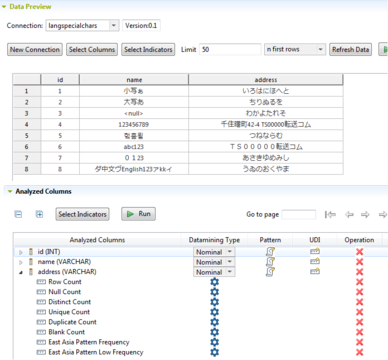The analysis results of the East Asia Pattern Low Frequency indicator will look like the following: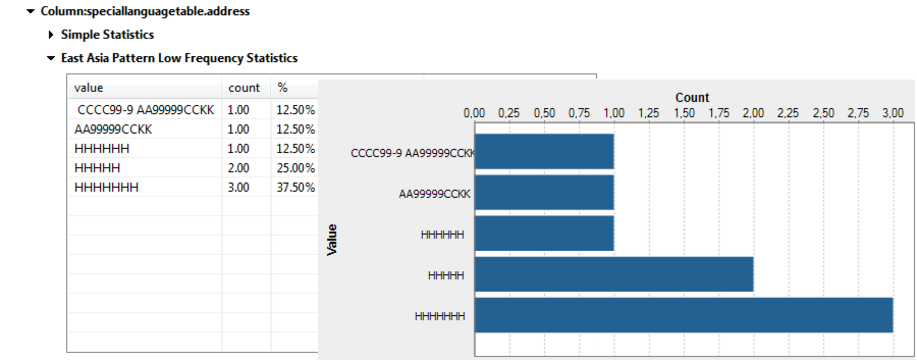These results give the number of the least frequent records for each distinct pattern. Some patterns have characters and numbers, while others have only characters. Patterns also have different lengths, so this shows that the address is not consistent and you may need to correct and clean it.

Phone number statistics

Indicators in this group count phone numbers. They return the count for each phone number format. They validate the phone formats using the org.talend.libraries.google.libphonumber library.

• Valid phone number count: computes the valid phone numbers.

• Possible phone number count: computes the supposed valid phone numbers.

• Valid region code number count: computes phone numbers with valid region code.

• Invalid region code count. computes phone numbers with invalid region code.

• Well formed national phone number count: computes well formatted national phone numbers.

• Well formed international phone number count: computes the international phone numbers that respect the international phone format (phone numbers that start with the country code) .

• Well formed E164 phone number count: computes the international phone numbers that respect the international phone format ( maximum of fifteen digits written with a + prefix.

• Format Phone Number Frequency: shows the results of the phone number count in a pie chart divided into sectors.

Simple statistics

They provide simple statistics on the number of records falling in certain categories including the number of rows, the number of null values, the number of distinct and unique values, the number of duplicates, or the number of blank fields.

• Blank Count: counts the number of blank rows. A "blank" is a non null textual data that contains only white space. Note that Oracle does not distinguish between the empty string and the null value.

• Default Value Count: counts the number of default values.

• Distinct Count: counts the number of distinct values of your column.

• Duplicate Count: counts the number of values appearing more than once. You have the relation: Duplicate count + Unique count = Distinct count. For example, a,a,a,a,b,b,c,d,e => 9 values, 5 distinct values, 3 unique values, 2 duplicate values.

• Null Count: counts the number of null rows.

• Row Count: counts the number of rows.

• Unique Count: counts the number of distinct values with only one occurrence. It is necessarily less or equal to Distinct counts.

Soundex frequency statistics

Indicators in this group use the Soundex algorithm built in the DBMS.

They index records by sounds. This way, records with the same pronunciation (only English pronunciation) are encoded to the same representation so that they can be matched despite minor differences in spelling.

• Soundex Frequency: computes the number of most frequent distinct records relative to the total number of records having the same pronunciation.

• Soundex Low Frequency: computes the number of less frequent distinct records relative to the total number of records having the same pronunciation.

Note

Due to some limitation in Teradata soundex implementation, you may not be able to drill down the results of profiling Teradata with this indicator. For further information, see the documentation on Talend Help Center (https://help.talend.com).

Summary statistics

They perform statistical analyses on numeric data, including the computation of location measures such as the median and the average, the computation of statistical dispersions such as the inter quartile range and the range.

• Mean: computes the average of the records.

• Median: computes the value separating the higher half of a sample, a population, or a probability distribution from the lower half.

• Inter quartile range: computes the difference between the third and first quartiles.

• Lower quartile (First quartile): computes the first quartile of data, that is the lowest 25% of data.

• Upper quartile (Third quartile): computes the third quartile of data, that is the highest 25% of data.

• Range: computes the difference between the maximum and minimum values.

When using the summary statistics indicators to profile a DB2 database, analysis results could be slightly different between Java and SQL engines. This is because indicators are computed differently depending on the database type, and also Talend uses special functions when working with Java.

Text statistics

You can use the text statistics indicators to analyze columns only if their data mining type is set to nominal in the analysis editor. Otherwise, these statistics will be grayed out in the [Indicator Selection] dialog box. For further information on the available data mining types, see Data mining types.

Text statistics analyze the characteristics of textual fields in the columns, including minimum, maximum and average length.

• Minimal Length: computes the minimal length of non-null and non-empty a text field.

• Maximal Length: computes the maximal length of non-null and non-empty a text field.

• Average Length: computes the average length of a non-null and non-empty field.

Other text indicators are available to count each of the above indicators with null values, with blank values or with null and blank values.

Null values will be counted as data of 0 length, that is to say the minimal length of null values is 0. This means that the Minimal Length With Null and the Maximal Length With Null will compute the minimal/maximal length of a text field including null values, that are considered to be 0-length text.

Blank values will be counted as regular data of 0 length, that is to say the minimal length of blank values is 0. This means that the Minimal Length With Blank and the Maximal Length With Blank will compute the minimal/maximal length of a text field including blank values.

The same will be applied for all average indicators.

For example, compute the length of textual fields in a column containing the following values, using all different types of text statistic indicators:

Value

Number of characters

Brayan

6

Ava

3

"_"

1

""

0

<null>

<null>

"__________"

10

Note

"_" represents a space character.

The results are as follows: